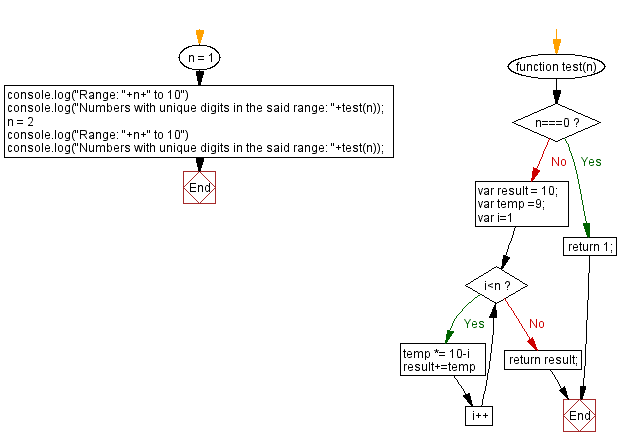# JavaScript - Count all the numbers with unique digits in a range

## JavaScript Math: Exercise-109 with Solution

Write a JavaScript program that accepts a number (n) and counts all numbers with unique digits of length p within a specified range.

Range: 0 <= p < 10n

Example:
When n = 1, numbers with unique digits (10) between 0 and 9 are 0, 1, 2, 3, 4, 5, 6, 7, 8, 9
When n = 2, numbers with unique digits (91) between 0 and 100 are 0,1, 2, 3, 4, 5, 6, 7, 8, 9, 10, 12, 13, 14, 15 …..99 except 11, 22, 33, 44, 55, 66, 77, 88 and 99.

Test Data:
(1) -> 10
(2) -> 91

Sample Solution:

HTML Code:

``````<!DOCTYPE html>
<html>
<meta charset="utf-8">
<title>JavaScript program to Count all numbers with unique digits in a range</title>
<body>

</body>
</html>
```
```

JavaScript Code:

``````function test(n) {
if(n===0){
return 1;
}
var result = 10;
var temp =9;
for(var i=1; i<n; i++){
temp *= 10-i
result+=temp
}
return result;
}
n = 1
console.log("Range: "+n+" to 10")
console.log("Numbers with unique digits in the said range: "+test(n));
n = 2
console.log("Range: "+n+" to 10")
console.log("Numbers with unique digits in the said range: "+test(n));
```
```

Sample Output:

```Range: 1 to 10
Numbers with unique digits in the said range: 10
Range: 2 to 10
Numbers with unique digits in the said range: 91
```

Flowchart:Live Demo:

See the Pen javascript-math-exercise-109 by w3resource (@w3resource) on CodePen.

Improve this sample solution and post your code through Disqus

What is the difficulty level of this exercise?

Test your Programming skills with w3resource's quiz.

﻿